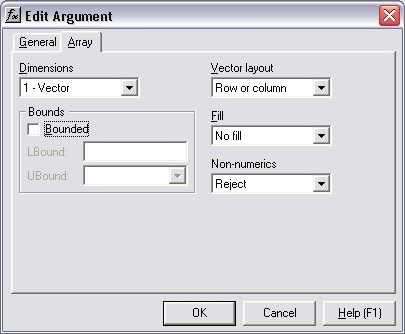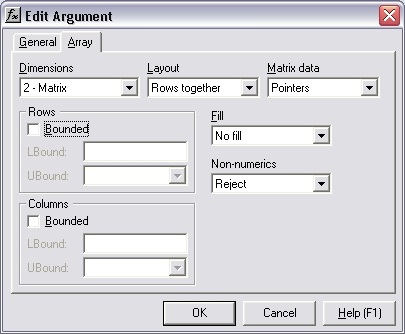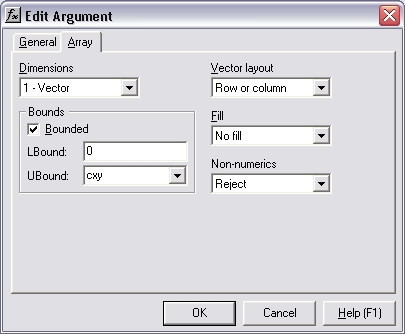# HOW TO: How to constrain the sizes of vector or matrix inputs

Reference: Q0034

The information in this article applies to:

• XLL+ for Visual Studio 2005 - 5.0
• XLL+ for Visual Studio .NET - 4.2, 4.3.1, 5.0
• XLL+ for Visual Studio 6 - 3, 4.1, 4.2, 4.3.1, 5.0

## HOW TO: How to constrain the sizes of vector or matrix inputs

### Questions

• How do I constrain a vector or matrix input to be a particular size?
• Can I force a vector input to contain a fixed number of cells?
• Can I force two or more vector inputs all to contain the same number of cells?
• How can I ensure that a matrix input is square?
• How can I ensure that one dimension of a matrix input matches the length of a vector input?

You can modify your arguments to be bounded input arrays. The XLL+ Function Wizard has a tab which lets you set the bounds of an array to be tied to a named variable (or to a constant). The code which is generated will automatically check that all matrix and vector inputs satisfy the constraints you apply.

### Using the Array tab of XLL+ Function Wizard's Argument Dialog

In the Xll+ Function Wizard, select the row containing a vector or matrix input, and click on the Dimensions cell. A button containing an ellipsis (...) will appear.Click on the button to show the Edit Argument dialog. (You can also use the Ctrl+E keyboard short-cut, or any of the methods listed under "Dimensions column" in the on-line help.)

Select the Array tab.By clicking on the Bounded check-box, you enable the LBound and UBound input boxes.

### Lower bounds

LBound, the lower bound, should normally be left unchanged, as `0`. LBound is only useful if you are passing the input to a library which expects arrays to be 1-based. If it is set to `1`, then an empty cell is prepended to the array when it is read in from Excel.

### Fixed upper bounds

UBound, the upper bound, can be set to a fixed value (e.g. `3`).

If you set UBound to a constant, then the code generated by the Function Wizard will check that the array is of the specified size, and will return an error value if it does not, e.g.:

```#Error: Expected 3 cells in x
#Error: Expected 5 rows in y
#Error: Expected 4 columns in z```

See FAQ #0035 for an example of a fixed size array.

### Named upper bounds

UBound, the upper bound can be set to the name of a C++ variable, referred to as a bounding variable.Type the name of a C++ variable (e.g. `cx`, or `sizeOfX`) into the UBound box. This will link the bounding variable to the size of the input array.

You don't need to declare the bounding variable in your code; the Wizard will do that for you.

If you use the same bounding variable for two inputs, then you constrain them both to be the same size. (Hint:- you can use the drop-down button in the UBound input box to get a list all bounding variables used by the current function.)

For example, you could constrain two vectors to be the same length by setting the UBound of each to the same variable. See FAQ #0036 for an example of two arrays that are constrained to be the same size.

Or you can force a matrix to be square by constraining the UBound of Rows and of Columns to the same variable. See FAQ #0037 for an example.

### Dimensions column

After you make changes to the bounding of an input variable, the new definition of the variable is displayed in the Dimensions column in the XLL+ Function Wizard.### Examples

The references listed below illustrate a number of cases where vector or matrix sizes are constrained.